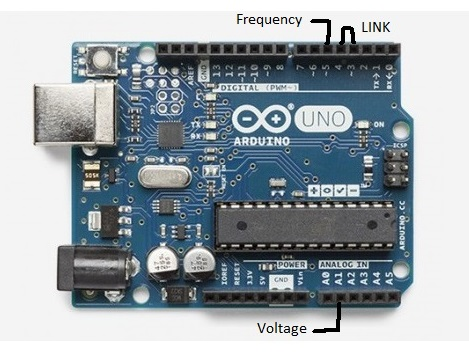Project tutorial

# Frequency Counter for PC

Arduino Uno used as a voltage meter and a 6 MHz frequency counter displayed on PC.

• 18,957 views
• 28 respects

## Apps and online services

 VB6 Software

The Arduino generates an accurate 1 second time base for the counter by cascading timer0 and timer2. The link between digital inputs 3 and 4 connects the output of timer2, 250 Hz, to input of timer0. The software waits for the output of timer0 to go positive to start the count of frequency input to timer1. Timer1 is a 16 bits timer, it overflows at the count of 2 power of 16, that in turn advances overFlow register. At the end of the 1 second the 16 bit register is recorded. The Arduino then sends to the PC via the USB cable and the Com Port 6 bytes of data.

The Arduino has to be connected to the PC first and then the VB6 app started. The app searches for the Com Port by sending a byte and checking if the byte is echoed. It takes a few seconds. The app has to be switched off when the Arduino IDE programs the Arduino Uno.

The frequency input is TTL signal levels, for low signal a video amp has to be added. The specs recommend input up to 6 MHz, I tested it at 2 MHz.

The app and VB6 code can be downloaded from my website: http://www.moty22.co.uk/android.php

## Code

##### vf meterC/C++
```/*
* Counter
* Created: 12/08/2017 23:34:47
*  Author: moty22.co.uk
*/

byte inByte=0, overF=0;
unsigned int freq=0;

// the setup function runs once when you press reset or power the board
void setup() {
// initialize serial communication at 9600 bits per second:
Serial.begin(9600);

//1 Hz timer
OCR0A = 249;
TCCR0A = _BV(WGM00) | _BV(WGM01) | _BV(COM0A0); //
TCCR0B = _BV(WGM02) | _BV(CS02) | _BV(CS01);  //  PWM mode, input T0 pin D4
pinMode(6, OUTPUT);

//250 Hz - timer2
OCR2A =249;
OCR2B = 125;
TCCR2A = _BV(COM2B1) | _BV(COM2B0) | _BV(WGM21) | _BV(WGM20);  //output B in phase, fast PWM mode
TCCR2B = _BV(WGM22) | _BV(CS22) | _BV(CS21); // set prescaler to 256 and start the timer
pinMode(3, OUTPUT);

//  counter input T1 pin D5
OCR1A = 32767;   //32768 counts
TCCR1A = _BV(WGM10) | _BV(WGM11) | _BV(COM1A0); //
TCCR1B =_BV(WGM12) | _BV(WGM13) | _BV(CS12) | _BV(CS11); //input pin D5
pinMode(9, OUTPUT);

//wait for connection from PC
while(Serial.available() == 0) {}
if(Serial.read() == 255){Serial.write(255);}     // if incoming byte=255 send 255.

}

// the loop function runs over and over again forever
void loop() {

if (Serial.available() > 0) {
if(inByte == 255){Serial.write(255);}

}
//counter

TIFR1 = _BV(OCF1A);    //reset int
OCR1A = 32767;
TCNT1=0;
overF=0;
if(TIFR1 & (1<<OCF1A)) {++overF; TIFR1 = _BV(OCF1A);}   //overflow
}
freq = TCNT1 ;

Serial.write(0);
Serial.write(overF);
Serial.write(highByte(freq));
Serial.write(lowByte(freq));

}
```

## Schematics#### Frequency Counter for Android

Project tutorial by moty

• 4,319 views
• 11 respects

#### Android Frequency Counter

Project tutorial by Projecter

• 2,984 views
• 5 respects

#### Counter

Project tutorial by moty

• 6,455 views
• 5 respects

#### Arduino Frequency Counter with 16×2 LCD Display

Project in progress by jasir

• 26,728 views
• 20 respects

#### Sampling Scope & Frequency Counter

Project showcase by Veldekiaan

• 5,305 views